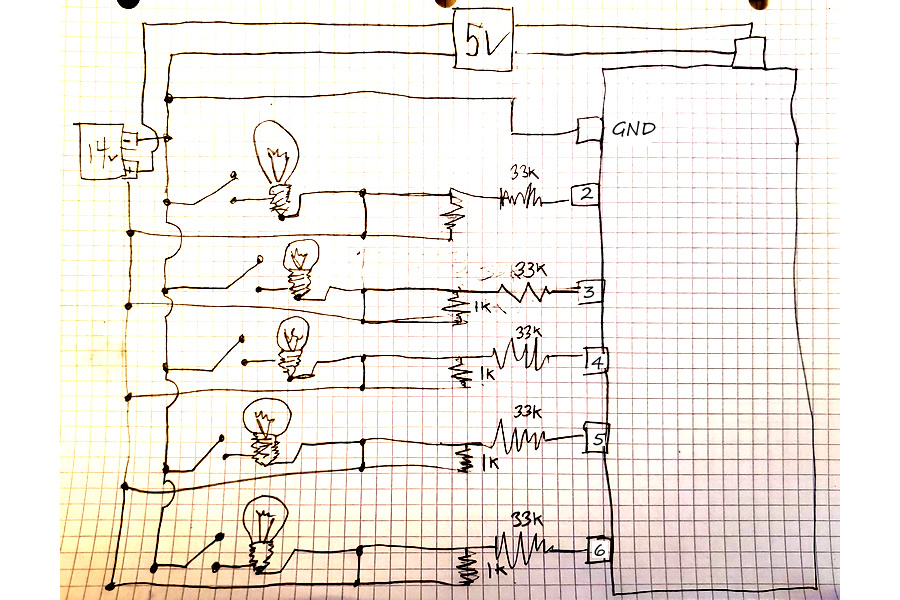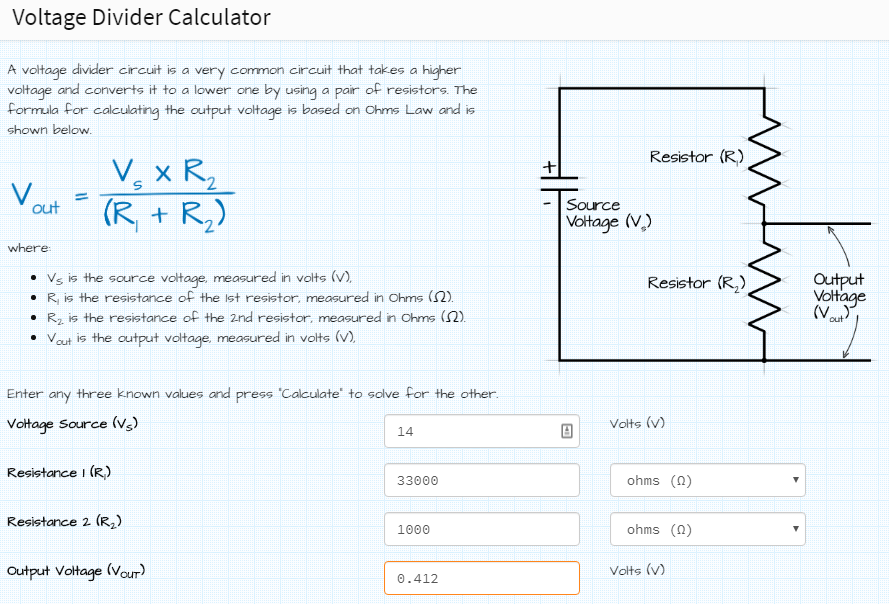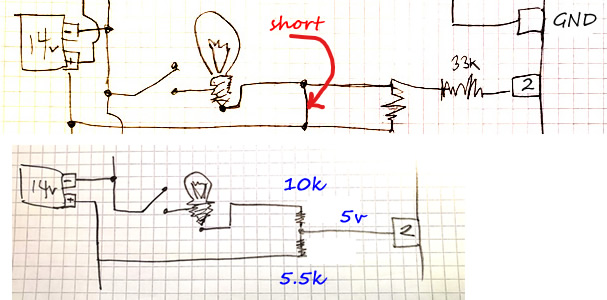# Correction: Using Voltage Divider to Monitor 14v circuit

I have a project where there are 5 lamps powered by a 14V transformer and I would like to monitor the on/off status of the lamps with an Arduino. One to Five lamps could be lit at any time. Does this diagram look correct? (330 Ohm resistors and 5.1v Zeners).Note: this was originally (inaccurately) titled: Using a Resistors/Zeners to Monitor 14v Lamps

Write a diagram, can be by hand, and attach. If the lamps are fed by AC, Your idea will not work. It will destroy Your Arduino.

Yes, the 14V is DC, powering the Arduino with a 9V buck converter. thx!

Your circuit will probably work but I can suggest improvements.

• Don't power the Arduino at 9V. Use a buck converter with a 5V output and feed 5V into the 5V input of the Arduino.
• 330R is far lower than necessary, and Zener diodes are not the usual way to do this. I suggest a voltage divider with, maybe, a 10k Ohm resistor between ground and the Arduino inputs (in place of the Zeners) and.... do I need to calculate the top resistor for you or can you do that? That's your homework!
• I'm not clear if you are using the analogue inputs as digital inputs (which is fine), or because you think they need to be analogue (which will work but is not necessary).

[EDIT] I just realised a problem with your circuit I didn't notice before: You are switching -ve to the lamps, so there is always a circuit through each lamp, its 330R resistor and Zener to 0V, so there will always be current flowing through the lamp filaments. Whether this is enough to make them glow I do not know. Doing it the way I suggest will very much reduce this current to a level that probably won't matter. Switching +ve will get rid of it completely.

As @PB says, 330 ohm is unneccessary low. 10k will probably do fine. Your idea with zener and resistor must be changed. The Arduino input must have a safe ground resistor, say some 27k - 47k. Else logic LOW might fail. Any helper that can check my post?

It seems you're trying to monitor the power supply to the lamps, not the lamps itself. To monitor the lamps (if they are actually working), you must measure current or light. Leo..

@Wawa I think something is wrong in the drawing. I see the contact fingers but is there a confusion mixing the in the relay coil? How/where are the coils?

Wawa: It seems you're trying to monitor the power supply to the lamps, not the lamps itself. To monitor the lamps (if they are actually working), you must measure current or light. Leo..

As he has it, and of he swaps Zeners for resistors, then if the bulb fails the input will be low, falsely indicating that the bulb is lit.

OP didn’t mention relays :o

With the current diagram, OP can detect if the lamps are there and not blown if… the lamps are off.
330ohm and a 5volt zener is a ‘dangerous’ design.
Max pin voltage is NOT 5volt, but VCC+0.5volt.
That’s 0.5volt if the Arduino happens to be off.
Leo…

Arduino off, max 0.5 volt. A really important situation. It's a bad design if the Arduino gets killed at a power loss.

I suggest a voltage divider with, maybe, a 10k Ohm resistor between ground and the Arduino inputs
You are switching -ve to the lamps, so there is always a circuit through each lamp

I see what you are saying, I will re-draw the circuit and do some homework on the voltage divider.
Thanks all! (Something is definitely wrong with the drawing.)

New games, new bets. Come back for the next round.

5 of these for \$10.00.Just a 33k resistor between the 14V point and the Arduino pin is all you need to keep the Arduino safe, relying on the clamping diodes.

The 330Ω ones are too small, and may indeed destroy the pins.

So the original circuit is a bit curious as it will read LOW if the lamp is on or else if it has burnt out when switched off. :astonished:

The proper values would be a 10k (or greater) resistor and a 4.7 V Zener, not a 5.1 V which is entirely useless.This (hopefully) addresses the design with a Voltage Divider that uses a 33K ohm resistor with a 1K ohm resistor. Which (I believe) will give the Arduino a ~0.4 signal. Also the signal is now on the negative leg of the circuit which means the Arduino will not be getting a signal unless a switch closes. I will monitor these on Digital pins.

For my application, I do not need to monitor the actual lamps, I need know if the circuit closes. (I now realize my original post title is technically inaccurate. @wawa and @bluejets are correct.)

1) Am I doing the Voltage Divider correctly? I used http://www.ohmslawcalculator.com/voltage-divider-calculator to calculate the values. Does the position of the 33K / 1K matter? Can they be swapped without consequence?2) Help me understand the 5V power suggestion... why should I not power the Arduino w/ 9V at the power jack (7 - 12V)?

Erm... Nice try and you are thinking along the right lines, but your 1k Ohm resistors all have short circuits across them, so do nothing.

You are looking for a divider that will give about 5V when there is 14V on the top resistor, not about 0V5. I am curious to know why you thought ~0V5 was the appropriator voltage.

You will notice I am not telling you the answer to your problem, this is because I want you to have the pleasure that comes with working it out for yourself :-)

The reason for not powering it from a higher voltage than 5V whenever possible is that the Arduino has a built in voltage regulator, which is a linear device, which means it converts the excess voltage into heat, so it wastes it. Worse, it has a very small heat sink to get rid of the waste heat, so is prone to over heating. As the amount of heat is proportional to the excess voltage the higher the voltage the more power is wasted as heat and the hotter the regulator gets. If you have a buck converter capable of producing the 5V you need directly then it makes sense to bypass the on board linear converter completely and just feed in 5V. A buck converter is a switching device and is much more efficient than a linear regulator. It essentially swaps voltage for current, less a bit of waste as nothing is perfect, as a result a buck converter wastes far less energy than a linear regulator.

Ah I see the short... ok here's a revision...As far as the 0.5v I guess I was confused by this comment...

@railroader "Arduino off, max 0.5 volt. A really important situation. It's a bad design if the Arduino gets killed at a power loss."

1) Is this 0.5v limitation because the previous design was monitoring the bulb by closing the circuit through the bulb... so THAT design would need to only trickle 0.5 volt... or it would harm the Arduino when the Arduino was off?

Since this circuit has 0 volts when the switch is open, I can allow 5v to the Arduino when the circuit closes? If this is the case and using your 10K suggestion... I came up with a 5.5K resistor for the other side. Based on this drawing... I'm sending the + into the Arduino.

2) Does it matter if I monitor the (+) side vs the (-)?

3) In my drawing 5.5k resistor would be R1 and the 10K would be R2 (closest to ground) does the larger value need to be R1? Does it matter?

On the 5V power supply... 4) Should Not use the barrel connector because 5v is too low? 5) Can I use the 5v pin (leaving the USB empty) so I can plug in my laptop for coding? Or do I need to feed the 5v into the USB and swap plugs when coding?

In case the Arduino looses its power, maximum safe voltage into any pin is 0.5 volt. Sorry but Your diagram will not let the bulb shine. I made a drawing but I don't know how to get it posted. Can TOU TELL ME?

Maybe I found out.